Select Page

In one of the previous articles, we explored image classification as one of the most common computer vision problems. However, this type of problem is not applicable to more complex projects, like let’s say self-driving cars. Problems from the real world usually include detecting some objects in the image or a video, preferably in real-time.

The human visual system is like that. We can take a quick look at an image and instantly know what objects are in the image, where they are, and how they interact. Our visual system is fast and accurate, allowing us to perform complex tasks with little conscious thought.

Are you afraid that AI might take your job? Make sure you are the one who is building it.

STAY RELEVANT IN THE RISING AI INDUSTRY! 🖖

In computer vision, the output of the object detection solution is not just the class of the object in the image. These systems are able to detect where objects are in the image and draw so-called a bounding box around it. Also, they provide a prediction of the class of the object in the image and confidence about that prediction.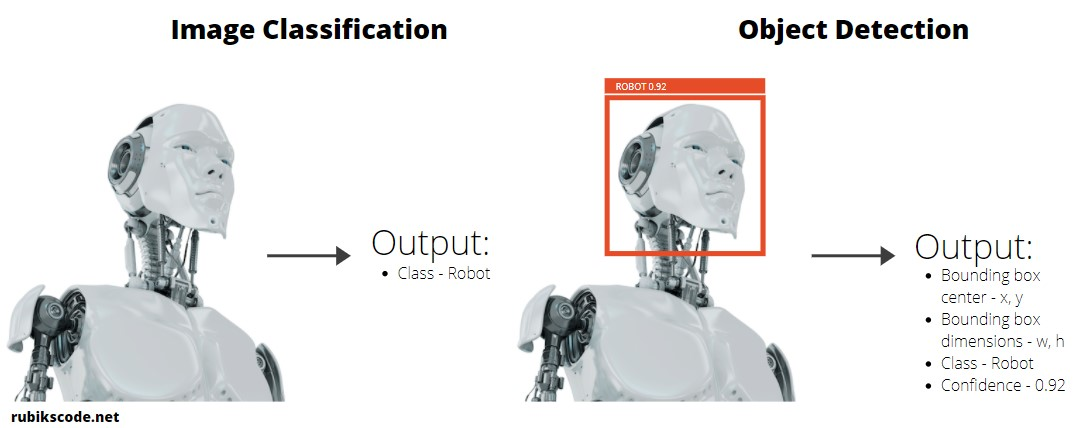Back in 2015. a shiny new architecture YOLO changed the industry and since then it became industry standard. Its acronym comes from the pun “You only look once” because this architecture simplified the process of object detection. The solutions that came before, like R-CNN, were usually “two-pass detectors”.

They first detected the regions where the objects might be and then classify them. YOLO is a single neural network that does that in one pass, thus the pun “You Only Look Once”. Here is what we explore in this article:

## 1. Prerequisites and Data

The implementations provided here are done in C#, and we use the latest .NET 5. So make sure that you have installed this SDK. If you are using Visual Studio this comes with version 16.8.3. Also, make sure that you have installed the following packages:

``````\$ dotnet add package Microsoft.ML

You can do the same from the Package Manager Console:

``````Install-Package Microsoft.ML
Install-Package Microsoft.ML.ImageAnalytics
Install-Package Microsoft.ML.OnnxRuntime
Install-Package Microsoft.ML.OnnxTransformer``````

You can do a similar thing using Visual Studio’s Manage NuGetPackage option: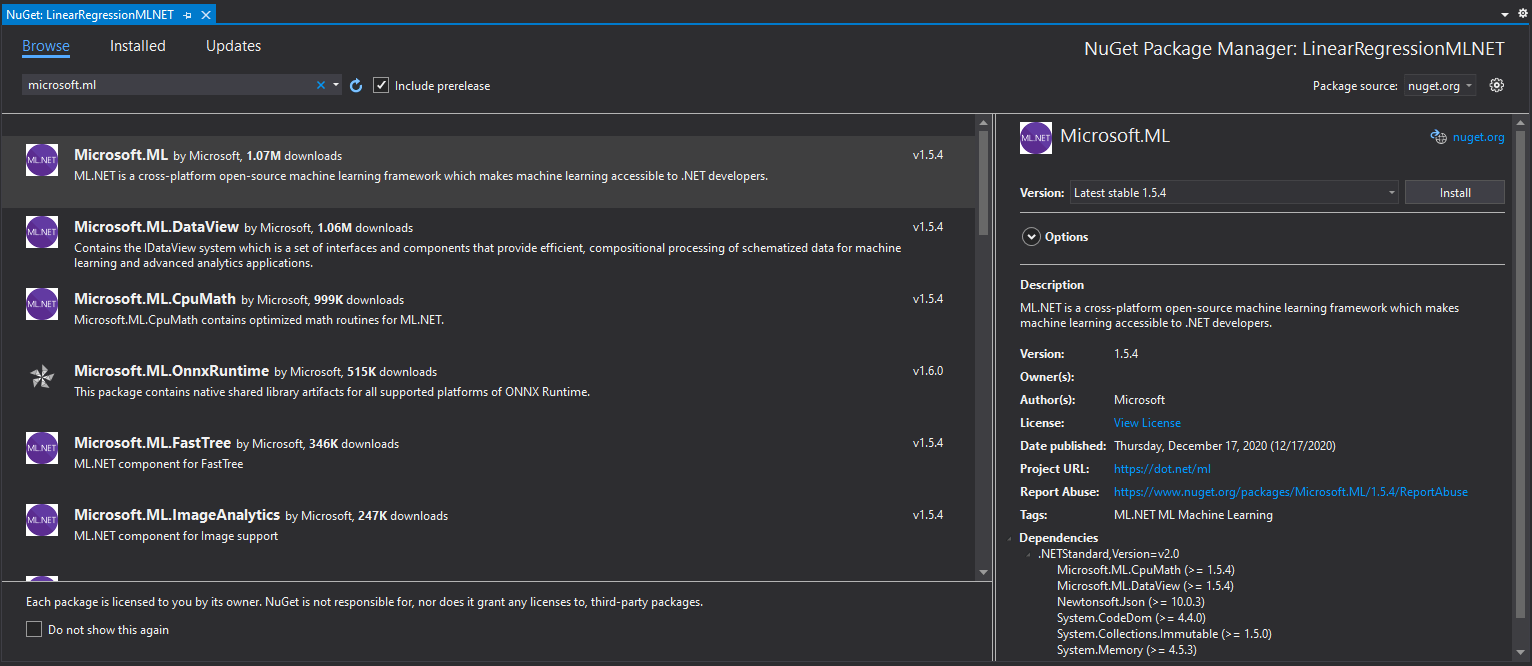If you need to catch up with the basics of machine learning with ML.NET check out this article.

Regarding the data, we use some random images from the internet. Feel free to use any images from the web that have categories used in this guide.

## 2. YOLO Approach

Let’s first describe how the first version of YOLO works. Then in the next section, we focus on improvements that other versions of YOLO introduce. As we mentioned, YOLO is a convolutional network that simultaneously predicts multiple bounding boxes and class probabilities for those boxes. So, how it does that?

In essence, YOLO divides the input image into an Sxgrid. If the object is in the center of the grid cell, then that grid cell should detect that object. This is done by predicting B bounding boxes and confidence scores within that grid cell. Each bounding box is defined by a five-element tuple (x, y, h, w, confidence). Coordinates (x, y) are coordinates of the center, while w and h are relative width and height.Confidence is the probability that a defined bounding box contains an object multiplied by intersection over union (IOU) between the predicted box and the ground truth. Apart from bounding boxes, each grid cell also predicts  conditional probabilities – Pr(Class i | Object).

In the next step, these conditional probabilities are multiplied with confidence for the bounding boxes to get all the bounding boxes weighted by their actual probabilities of containing that object. Finally, to get a single best detection for an object, we perform a Non-Max Suppression. This technique, removes all low confidence values and picks the best one.

## 3. YOLO Versions

That is in a nutshell how the first version of YOLO functions. However, this first version is extended over the years with new concepts and changes in the architecture. However, the core principles remained. Let’s check out which improvements each of the versions brought.

### 3.1 YOLOv2

So-called “Better, Faster, Stronger YOLO” brought many improvements. It introduced many features for which YOLO is known and loved. It brought performance improvements, introduced anchors and multi-scale training. Then this architecture is trained on a combination of ImageNet and COCO dataset so it is able to recognize 9000 classes of objects – YOLO9000.

Probably the most noticeable change is the introduction of Anchor boxes. Older concepts like Faster R-CNN used the concept of pre-given anchor boxes to predict bounding boxes for objects. Basically, they didn’t use regression to predict x, y, w and h, like YOLOv1 did. They used 3 different scales and 3 different aspect ratios to compute the offsets for these pre-given anchor boxes. Then they predicted boxes using that offset.This way algorithm needs to learn offset and selected size, and it doesn’t need to learn coordinates and dimensions of the bounding box. YOLOv2 goes one step further. Instead of using predefined anchor boxes, it uses bounding boxes of training data. Then it runs K-Means Clustering on them and picks set of dimension clusters that are applicable for the concrete problem.

YOLOv2 also introduced multi-scale training. This means that the network is randomly resized during the training process in the multiples of 32. This seems to have increased the performance of YOLOv2.

Finally, this version used WordTree, a specially tailored dataset using a combination of COCO and ImageNet. To combine these two datasets together, a tree structure is implemented with hierarchies like wordnet. So, instead of having a single SoftMax deciding which class is in the image, the whole tree is used. This way YOLOv2 is able to classify more than initial 80 classes.

### 3.2 YOLOv3

YOLOv3 is the star of the YOLOs. With the improvements this version brought, YOLOv3 became the most popular architecture for object detection. It focused on improving existing concepts further, nothing groundbreaking, but still cool.

Overall some of the improvements are

• More bounding boxes per image – YOLOv3 predicts 10x more bounding boxes than YOLOv2 in 3 different scales.
• Class Prediction – Instead of SoftMax YOLOv3  uses independent logistic classifiers with binary cross-entropy loss.
• New Feature Extractor – YOLOv3 uses a new convolutional neural network with 53 layers (Darknet-53) for feature extraction .which is more powerful then Darknet-19 (used in YOLOv2), ResNet-101 and ResNet-152.### 3.3 YOLOv4 & YOLOv5

There is a lot of controversy surrounding YOLOv4 and YOLOv5.  This all started when the original author of YOLO Joseph Redmon announced that he has stopped his research in computer vision back in February 2020. He stated that this was due to several concerns regarding the potential negative impact of his work.

However, in April 2020 YOLOv4 paper by Alexey Bochkovskiy was released. The work was continued on a fork of the main repository. Authors introduce two terms Bag of freebies (BOF) and Bag of specials (BOS). Bag of freebies refers to the methods that affect training strategy.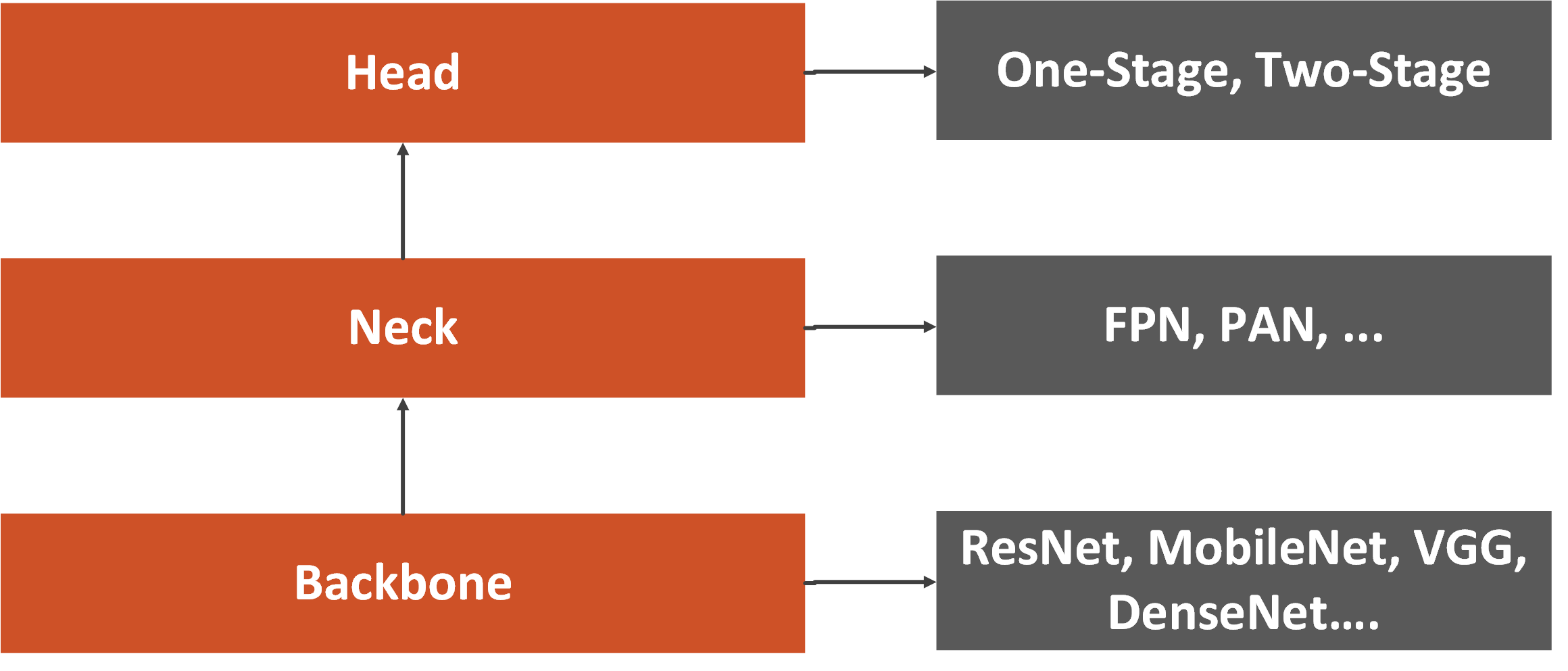One such method is data augmentation, which is used to increase the variability of the input images and make the model has higher robustness. Other methods that could be considered as Bag of freebies are random erase, CutOut, grid mask, DropOut, DropConnect, etc. All these methods temper with the input images and/or feature maps and remove bias from input data.

Finally, Bag of freebies could be some objective functions like Bounding Box (BBox). Bag of specials is post-processing modules and methods that do increase the inference cost but improve the accuracy of object detection as well. These can be any methods enhancing certain features of a model. For example, that can be enlarging receptive field, introducing attention mechanism, or strengthening feature integration capability, etc.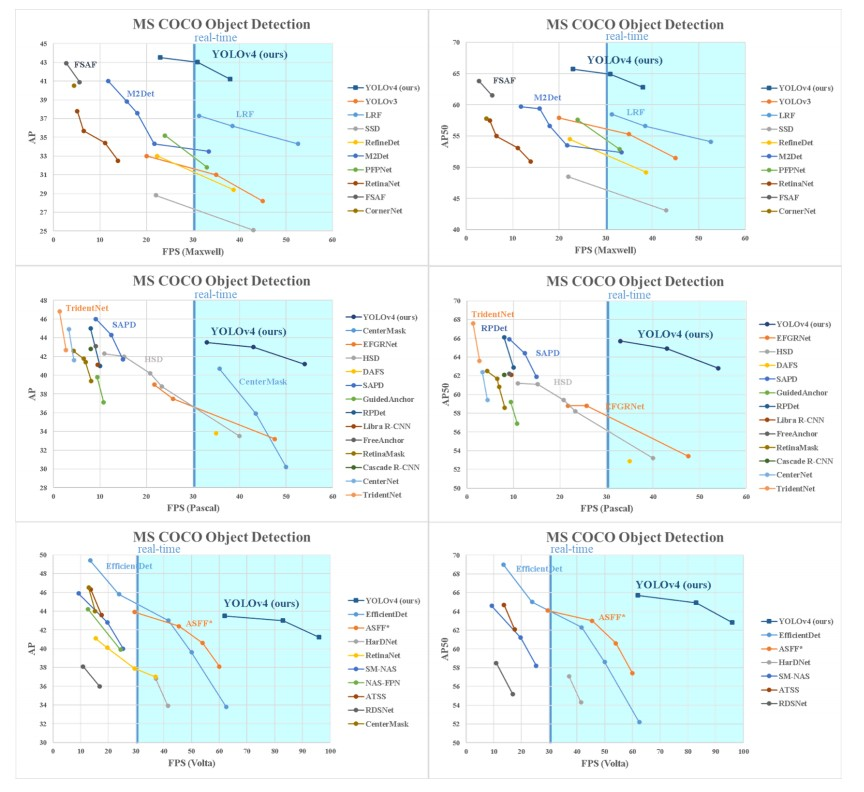Based on all of these, the architecture of YOLOv4 consists of the following parts:
Backbone: CSPDarknet53 – Cross Stage Partial Network minimizing required heavy inference computations from the network architecture perspective.
Neck: Spatial Pyramid Pooling – SPP (so object-detector can receive images of arbitrary size/scale) and Path Aggregation Network – PAN (boosting information flow in proposal-based instance segmentation framework)
Bag of Freebies (BoF) for backbone: CutMix and Mosaic data augmentation, DropBlock regularization, Class label smoothing
Bag of Specials (BoS) for backbone: Mish activation, Cross-stage partial connections (CSP), Multi-input weighted residual connections (MiWRC)
Bag of Freebies (BoF) for detector: CIoU-loss, CmBN, DropBlock regularization, Mosaic data augmentation, Self-Adversarial Training, Eliminate grid sensitivity, Using multiple anchors for single ground truth, Cosine annealing scheduler, Optimal hyperparameters, Random training shapes
Bag of Specials (BoS) for detector: Mish activation, SPP-block, SAM-block, PAN path-aggregation block, DIoU-NMS

We never got paper for YOLOv5.  This version was built by Glenn Jocher, who is well known for creating the popular PyTorch implementation of YOLO v3. This version is completely different from the previous versions and it uses PyTorch implementation and not original Darknet architecture.

## 4. ONNX Models

Before we dive into the implementation of object detection application with ML.NET we need to cover one more theoretical thing. That is the Open Neural Network Exchange (ONNX) file format. This file format is an open-source format for AI models and it supports interoperability between frameworks.

Basically, you can train a model in one machine learning framework like PyTorch, save it and convert it into ONNX format. Then you can consume that ONNX model in a different framework like ML.NET. That is exactly what we do in this tutorial. You can find more information on the ONNX website.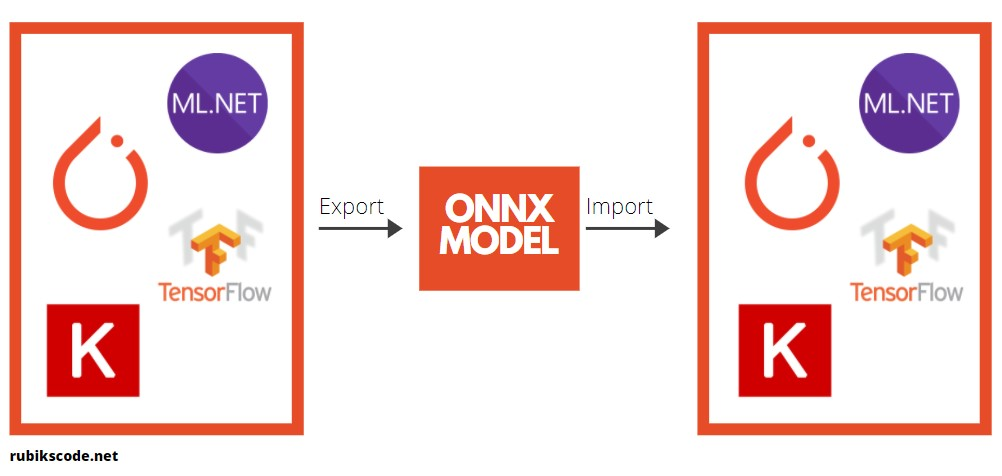In this tutorial, we use the pre-trained YOLOv4 model. This model is available here. In essence, we import this model into ML.NET and run it within our application.One very interesting and useful thing we can do with the ONNX model is that there are a bunch of tools we can use for a visual representation of the model. This is very useful when we use pre-trained models as we do in this tutorial.

We often need to know the names of input and output layers, and this kind of tool is good for that. So, once we download the YOLOv4 model, we can load it with one of the tools for visual representation. In this guide, we use Netron and here is just the part of the output:After all, YOLOv4 is a big model. However, we can observe the output of this model, since we need to reflect it in our application: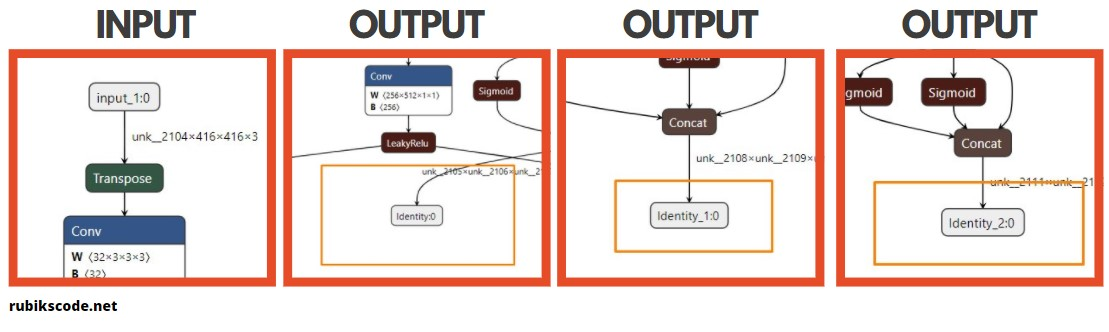We can notice input named “input_1:0” and that the outputs are named “Identity:0”, “Identity1:0” and “Identity2:0”, respectivly.

## 5. Implementation with ML.NET

Ok, let’s start with the high-level project architecture. In essence, we use Trainer to load a pre-trained model. Then we run predictions using Predictor and finally use Drawer to write those outputs as a .jpg file. Outputs should contain bounding boxes around objects, class of the object and confidence score.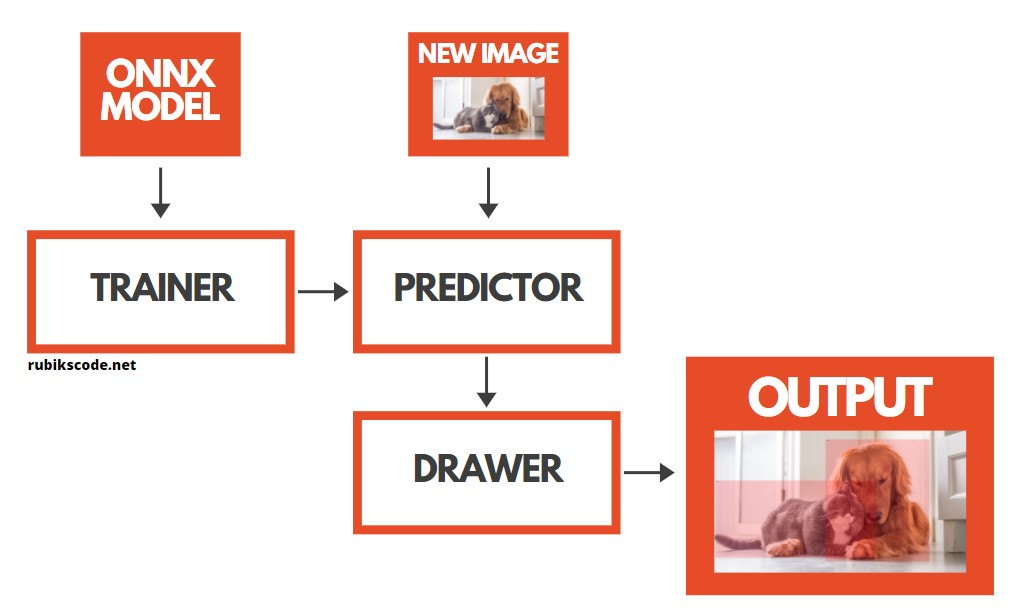To implement this architecture we created project structure that looks like this:Here in the Assets folder, you can find the downloaded .onnx model and folder with images on which we want to perform the object detection. Here is of those images: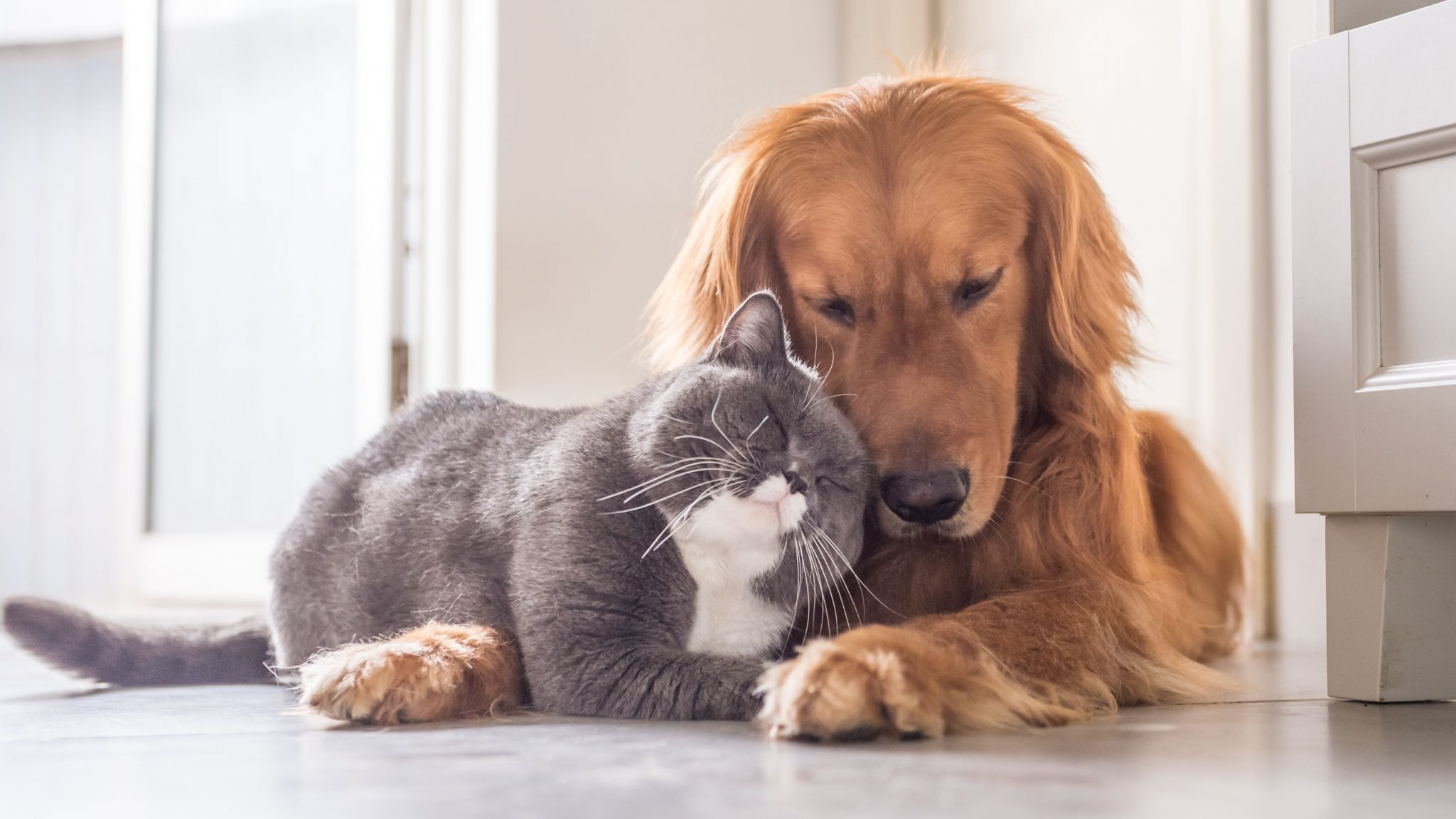Within the Assets folder, there is the Output sub-folder which will later contain the output of the processing. The Machine Learning folder contains all the necessary code that we use in this application. The Trainer and Predictor classes are there, just like the classes which are modeling data. In the separate folder, we can find DrawResults helper class.

### 5.1 Data Models

You may notice that in the DataModel folder we have three classes. This is a bit different than the classes we had in previous and similar tutorials. The ImageData class is there to represent the input:

``````using Microsoft.ML.Data;
using Microsoft.ML.Transforms.Image;
using System.Drawing;

namespace YoloV4MlNet.MachineLearning.DataModel
{
public class ImageData
{
[ColumnName("image")]
[ImageType(416, 416)]
public Bitmap Image { get; set; }

[ColumnName("width")]
public float ImageWidth => Image.Width;

[ColumnName("height")]
public float ImageHeight => Image.Height;
}
}
``````

However, the real fun happens in the ImagePrediction class. This class is more complicated than anything we saw so far in the previous tutorials. This class handles output from the YOLO model, does necessary post-processing and returns an object of Result class, a class which contains Bounding Box, Label and Confidence. Let’s take a look at the ImagePrediction class:

``````using Microsoft.ML.Data;
using System;
using System.Collections.Generic;
using System.Diagnostics;
using System.Linq;

namespace YoloV4MlNet.MachineLearning.DataModel
{
public class ImagePrediction
{
private readonly float[][][] ANCHORS = new float[][][]
{
new float[][] { new float[] { 12, 16 }, new float[] { 19, 36 }, new float[] { 40, 28 } },
new float[][] { new float[] { 36, 75 }, new float[] { 76, 55 }, new float[] { 72, 146 } },
new float[][] { new float[] { 142, 110 }, new float[] { 192, 243 }, new float[] { 459, 401 } }
};

// Read more on YOLO configuration here:
// https://github.com/hunglc007/tensorflow-yolov4-tflite/blob/9f16748aa3f45ff240608da4bd9b1216a29127f5/core/config.py#L18
// https://github.com/hunglc007/tensorflow-yolov4-tflite/blob/9f16748aa3f45ff240608da4bd9b1216a29127f5/core/config.py#L20

private readonly float[] STRIDES = new float[] { 8, 16, 32 };
private readonly float[] XYSCALE = new float[] { 1.2f, 1.1f, 1.05f };
private readonly int[] SHAPES = new int[] { 52, 26, 13 };

private const int _anchorsCount = 3;
private const float _scoreThreshold = 0.5f;
private const float _iouThreshold = 0.5f;

/// <summary>
/// Output - Identity
/// </summary>
[VectorType(1, 52, 52, 3, 85)]
[ColumnName("Identity:0")]
public float[] Identity { get; set; }

/// <summary>
/// Output - Identity 1:0
/// </summary>
[VectorType(1, 26, 26, 3, 85)]
[ColumnName("Identity_1:0")]
public float[] Identity1 { get; set; }

/// <summary>
/// Output - Identity 2:0
/// </summary>
[VectorType(1, 13, 13, 3, 85)]
[ColumnName("Identity_2:0")]
public float[] Identity2 { get; set; }

[ColumnName("width")]
public float ImageWidth { get; set; }

[ColumnName("height")]
public float ImageHeight { get; set; }

{
var postProcesssedBoundingBoxes = PostProcessBoundingBoxes(new[] { Identity, Identity1, Identity2 }, categories.Length);
return NMS(postProcesssedBoundingBoxes, categories);
}

/// <summary>
/// This method is postprocess_bbbox()
/// Ported from https://github.com/onnx/models/tree/master/vision/object_detection_segmentation/yolov4#postprocessing-steps
/// Thans to the help of: https://github.com/BobLd/YOLOv4MLNet/blob/master/YOLOv4MLNet/DataStructures/YoloV4Prediction.cs
/// </summary>
/// <returns></returns>
private List<float[]> PostProcessBoundingBoxes(float[][] results, int classesCount)
{
List<float[]> postProcesssedResults = new List<float[]>();

for (int i = 0; i < results.Length; i++)
{
var pred = results[i];
var outputSize = SHAPES[i];

for (int boxY = 0; boxY < outputSize; boxY++)
{
for (int boxX = 0; boxX < outputSize; boxX++)
{
for (int a = 0; a < _anchorsCount; a++)
{
var offset = (boxY * outputSize * (classesCount + 5) * _anchorsCount) + (boxX * (classesCount + 5) * _anchorsCount) + a * (classesCount + 5);
var predBbox = pred.Skip(offset).Take(classesCount + 5).ToArray();

var predXywh = predBbox.Take(4).ToArray();
var predConf = predBbox;
var predProb = predBbox.Skip(5).ToArray();

var rawDx = predXywh;
var rawDy = predXywh;
var rawDw = predXywh;
var rawDh = predXywh;

float predX = ((Sigmoid(rawDx) * XYSCALE[i]) - 0.5f * (XYSCALE[i] - 1) + boxX) * STRIDES[i];
float predY = ((Sigmoid(rawDy) * XYSCALE[i]) - 0.5f * (XYSCALE[i] - 1) + boxY) * STRIDES[i];
float predW = (float)Math.Exp(rawDw) * ANCHORS[i][a];
float predH = (float)Math.Exp(rawDh) * ANCHORS[i][a];

// (x, y, w, h) --> (xmin, ymin, xmax, ymax)
float predX1 = predX - predW * 0.5f;
float predY1 = predY - predH * 0.5f;
float predX2 = predX + predW * 0.5f;
float predY2 = predY + predH * 0.5f;

// (xmin, ymin, xmax, ymax) -> (xmin_org, ymin_org, xmax_org, ymax_org)
float org_h = ImageHeight;
float org_w = ImageWidth;

float inputSize = 416f;
float resizeRatio = Math.Min(inputSize / org_w, inputSize / org_h);
float dw = (inputSize - resizeRatio * org_w) / 2f;
float dh = (inputSize - resizeRatio * org_h) / 2f;

var orgX1 = 1f * (predX1 - dw) / resizeRatio;
var orgX2 = 1f * (predX2 - dw) / resizeRatio;
var orgY1 = 1f * (predY1 - dh) / resizeRatio;
var orgY2 = 1f * (predY2 - dh) / resizeRatio;

// Clip boxes that are out of range
orgX1 = Math.Max(orgX1, 0);
orgY1 = Math.Max(orgY1, 0);
orgX2 = Math.Min(orgX2, org_w - 1);
orgY2 = Math.Min(orgY2, org_h - 1);

if (orgX1 > orgX2 || orgY1 > orgY2)
{
continue;
}

// Discard boxes with low scores
var scores = predProb.Select(p => p * predConf).ToList();

float scoreMaxCat = scores.Max();
if (scoreMaxCat > _scoreThreshold)
{
postProcesssedResults.Add(new float[] { orgX1, orgY1, orgX2, orgY2, scoreMaxCat, scores.IndexOf(scoreMaxCat) });
}
}
}
}
}

return postProcesssedResults;
}

/// <summary>
/// Performs Non-Maximum Suppression.
/// </summary>
/// <returns>List of Results</returns>
private List<Result> NMS(List<float[]> postProcesssedBoundingBoxes, string[] categories)
{
postProcesssedBoundingBoxes = postProcesssedBoundingBoxes.OrderByDescending(x => x).ToList();
var resultsNms = new List<Result>();

int counter = 0;
while (counter < postProcesssedBoundingBoxes.Count)
{
var result = postProcesssedBoundingBoxes[counter];
if (result == null)
{
counter++;
continue;
}

var confidence = result;
string label = categories[(int)result];

postProcesssedBoundingBoxes[counter] = null;

var iou = postProcesssedBoundingBoxes.Select(bbox => bbox == null ? float.NaN : BoxIoU(result, bbox)).ToList();

for (int i = 0; i < iou.Count; i++)
{
if (float.IsNaN(iou[i]))
{
continue;
}

if (iou[i] > _iouThreshold)
{
postProcesssedBoundingBoxes[i] = null;
}
}
counter++;
}

return resultsNms;
}

/// <summary>
/// Intersection-over-union (Jaccard index) of boxes.
/// </summary>
private float BoxIoU(float[] boxes1, float[] boxes2)
{
var area1 = GetBoxArea(boxes1);
var area2 = GetBoxArea(boxes2);

var dx = Math.Max(0, Math.Min(boxes1, boxes2) - Math.Max(boxes1, boxes2));
var dy = Math.Max(0, Math.Min(boxes1, boxes2) - Math.Max(boxes1, boxes2));

return (dx * dy) / (area1 + area2 - (dx * dy));
}

private float GetBoxArea(float[] box)
{
return (box - box) * (box - box);
}

private float Sigmoid(float x)
{
return 1f / (1f + (float)Math.Exp(-x));
}
}
}
``````

It is one large class. In the beginning, we initialize anchors, stride and scale. For more information about how to configure and initialize YOLO, take a look here and here. We also initialize thresholds and outputs. Note that here, for the ColumnName we use names that we saw in the graphic representation of YOLO.

Also, here we can find GetResult method, which is the only public method from this class. Here we first post-process bounding boxes that we get from YOLOv4, which means we clip boxes that are out of range and discard boxes with a low score, etc. Then we perform Non-Maximum Suppression. As the output, we get the list of Result objects. The Result class is simple:

``````namespace YoloV4MlNet.MachineLearning.DataModel
{
public class Result
{
/// <summary>
/// x1, y1, x2, y2 in page coordinates.
/// </summary>
public float[] BoundingBox { get; }

/// <summary>
/// The Bounding box category.
/// </summary>
public string Label { get; }

/// <summary>
/// Confidence level.
/// </summary>
public float Confidence { get; }

public Result(float[] boundingBox, string label, float confidence)
{
BoundingBox = boundingBox;
Label = label;
Confidence = confidence;
}
}
}
``````

### 5.2 Trainer

The Trainer class is quite simple, it has only one method BuildAndTrain which uses the path to the pre-trained model.

``````using Microsoft.ML;
using System.Collections.Generic;
using System.Linq;
using YoloV4MlNet.MachineLearning.DataModel;
using static Microsoft.ML.Transforms.Image.ImageResizingEstimator;

namespace YoloV4MlNet.MachineLearning
{
public class Trainer
{
private MLContext _mlContext;

public Trainer()
{
_mlContext = new MLContext();
}

public ITransformer BuildAndTrain(string yoloModelPath)
{
var pipeline = _mlContext.Transforms.ResizeImages(inputColumnName: "image",
outputColumnName: "input_1:0", imageWidth: 416, imageHeight: 416,
.Append(_mlContext.Transforms.ExtractPixels(outputColumnName: "input_1:0",
scaleImage: 1f / 255f,
interleavePixelColors: true))
.Append(_mlContext.Transforms.ApplyOnnxModel(
shapeDictionary: new Dictionary<string, int[]>()
{
{ "input_1:0", new[] { 1, 416, 416, 3 } },
{ "Identity:0", new[] { 1, 52, 52, 3, 85 } },
{ "Identity_1:0", new[] { 1, 26, 26, 3, 85 } },
{ "Identity_2:0", new[] { 1, 13, 13, 3, 85 } },
},
inputColumnNames: new[]
{
"input_1:0"
},
outputColumnNames: new[]
{
"Identity:0",
"Identity_1:0",
"Identity_2:0"
},
modelFile: yoloModelPath));

}

}
}
``````

In the mentioned method, we build the pipeline. First, we resize the image to 416×416. Then we normalize it, ie. we scale the image. At the end of the pipeline, we apply the ONNX model. Finally, we fit this model to empty data. We do this, so we can load the data schema, ie. to load the model.

### 5.3 Predictor

The Predictor class is even more simple. It receives a trained and loaded model and creates a prediction engine. Then it uses this prediction engine to create predictions on new images.

``````using Microsoft.ML;
using System.Drawing;
using YoloV4MlNet.MachineLearning.DataModel;

namespace YoloV4MlNet.MachineLearning
{
public class Predictor
{
private MLContext _mLContext;
private PredictionEngine<ImageData, ImagePrediction> _predictionEngine;

public Predictor(ITransformer trainedModel)
{
_mLContext = new MLContext();
_predictionEngine = _mLContext.Model
.CreatePredictionEngine<ImageData, ImagePrediction>(trainedModel);
}

public ImagePrediction Predict(Bitmap image)
{
return _predictionEngine.Predict(new ImageData() { Image = image });
}
}
}
``````

### 5.4 Drawer

The DrawResults static class is used to create the output image with bounding boxes.

``````using System.Collections.Generic;
using System.Drawing;
using System.IO;
using YoloV4MlNet.MachineLearning.DataModel;

namespace YoloV4MlNet.Drawer
{
public static class DrawResults
{
public static void DrawAndStore(string imageOutputFolder, string imageName,
{
using (var graphics = Graphics.FromImage(image))
{
foreach (var result in results)
{
var x1 = result.BoundingBox;
var y1 = result.BoundingBox;
var x2 = result.BoundingBox;
var y2 = result.BoundingBox;

graphics.DrawRectangle(Pens.Red, x1, y1, x2 - x1, y2 - y1);

using (var brushes = new SolidBrush(Color.FromArgb(50, Color.Red)))
{
graphics.FillRectangle(brushes, x1, y1, x2 - x1, y2 - y1);
}

graphics.DrawString(result.Label + " " + result.Confidence.ToString("0.00"),
new Font("Arial", 12), Brushes.Blue, new PointF(x1, y1));
}

image.Save(Path.Combine(imageOutputFolder, Path.ChangeExtension(imageName,"_yoloed"
+ Path.GetExtension(imageName))));
}
}
}
}
``````

### 5.5 Program

We put all this together in the Program file.

``````using System;
using System.Drawing;
using System.IO;
using YoloV4MlNet.Drawer;
using YoloV4MlNet.MachineLearning;

namespace YoloV4MlNet
{
class Program
{
private const string _modelPath = @"..\YoloV4MlNet\Assets\Model\yolov4.onnx";
private const string _imageFolder = @"..\YoloV4MlNet\Assets\Data";
private const string _imageOutputFolder = @"..\YoloV4MlNet\Assets\Output";
private static readonly string[] _classesNames = new string[] {
"person", "bicycle", "car", "motorbike", "aeroplane", "bus", "train", "truck", "boat", "traffic light", "fire hydrant", "stop sign", "parking meter",
"bench", "bird", "cat", "dog", "horse", "sheep", "cow", "elephant", "bear", "zebra", "giraffe", "backpack", "umbrella", "handbag", "tie", "suitcase",
"frisbee", "skis", "snowboard", "sports ball", "kite", "baseball bat", "baseball glove", "skateboard", "surfboard", "tennis racket", "bottle", "wine glass",
"cup", "fork", "knife", "spoon", "bowl", "banana", "apple", "sandwich", "orange", "broccoli", "carrot", "hot dog", "pizza", "donut", "cake", "chair", "sofa",
"pottedplant", "bed", "diningtable", "toilet", "tvmonitor", "laptop", "mouse", "remote", "keyboard", "cell phone", "microwave", "oven", "toaster", "sink",
"refrigerator", "book", "clock", "vase", "scissors", "teddy bear", "hair drier", "toothbrush" };

static void Main()
{
Directory.CreateDirectory(_imageOutputFolder);
var trainer = new Trainer();

Console.WriteLine("Build and train YOLO V4 model...");
var trainedModel = trainer.BuildAndTrain(_modelPath);

Console.WriteLine("Create predictor...");
var predictor = new Predictor(trainedModel);

Console.WriteLine("Run predictions on images...");

DirectoryInfo directoryInfo = new DirectoryInfo(_imageFolder);
FileInfo[] files = directoryInfo.GetFiles("*.jpg");

foreach (FileInfo file in files)
{
using (var image = new Bitmap(Image.FromFile(Path.Combine(_imageFolder, file.Name))))
{
var predict = predictor.Predict(image);
var results = predict.GetResults(_classesNames);

DrawResults.DrawAndStore(_imageOutputFolder, file.Name, results, image);
}
}

Console.WriteLine(\$"Check images in the output folder {_imageOutputFolder}...");
}
}
}
``````

First, we create the Output folder and the Trainer object. Then we load the model and create a Predictor object. Finally, we run the predictions on all the images from the Data folder and store them. The output in the console looks like this:

``````Build and train YOLO V4 model...
Create predictor...
Run predictions on images...
Check images in the output folder \YoloV4MlNet\YoloV4MlNet\Assets\Output...``````

And here are some output images: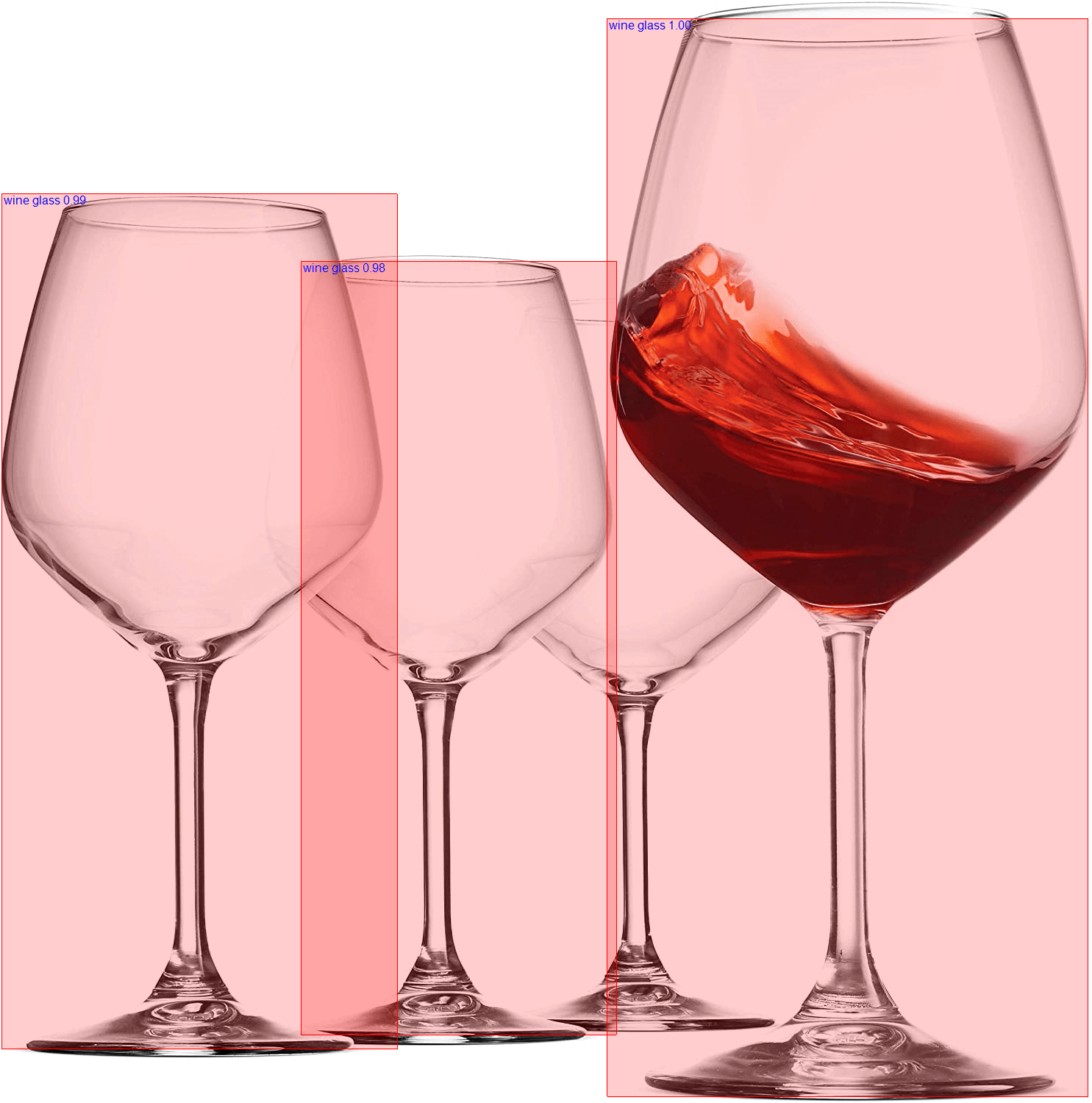## Conclusion

In this article, we learned how object detection works. To be more specific, we talked about how YOLO architecture works. We also had a chance to explore different versions of YOLO and to see what each of those architectures brought. Finally, we learned about ONNX model format and how we can use it with ML.NET.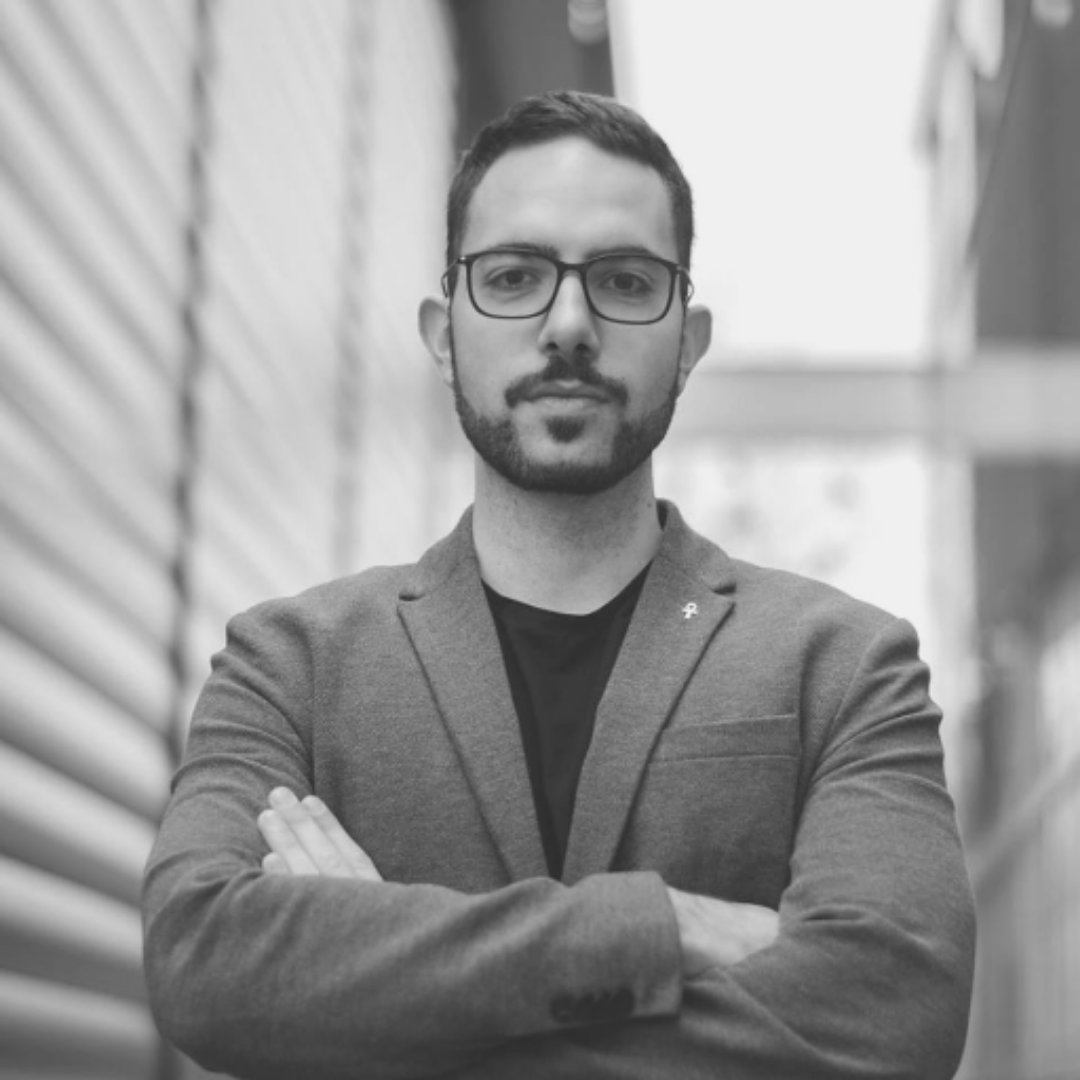#### Nikola M. Zivkovic

CAIO at Rubik's Code

Nikola M. Zivkovic a CAIO at Rubik’s Code and the author of book “Deep Learning for Programmers“. He is loves knowledge sharing, and he is experienced speaker. You can find him speaking at meetups, conferences and as a guest lecturer at the University of Novi Sad.

Rubik’s Code is a boutique data science and software service company with more than 10 years of experience in Machine Learning, Artificial Intelligence & Software development. Check out the services we provide.

Ultimate Guide to Machine Learning with Python

Everything from Python basics to the deployment of Machine Learning algorithms to production in one place.

Become a Machine Learning Superhero TODAY!